Visualization Library 2.0.0 A lightweight C++ OpenGL middleware for 2D/3D graphics VL     Star     Watch     Fork     Issue [Download] [Tutorials] [All Classes] [Grouped Classes]
Transparency and Polygon Depth Sorting Tutorial

In this tutorial you will learn how to perform primitive depth sorting in order to obtain correct transparencies.

Managing transparent objects in the correct way using OpenGL (but also DirectX) can be quite challenging at times. This is because in general, when rendering transparent objects, the order in which such objects are rendered affects the final result. Theoretically the problem could be solved by sorting back to front each single pixel that is generated by the rendering process. In practice this approach is too expensive to implement and for simple cases it is enough to sort front to back entire objects. This works well util the transparent objects in the scene are convex but when we add concave objects we will start noticing some artifacts. In this cases it is necessary to sort back to font the polygons of each object. When two transparent objects intersect each other, though, not even this approach yelds correct results in general. Fortunately this last case is relatively rare compared to the previous ones.

 Depth sorting on: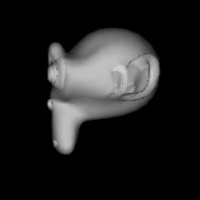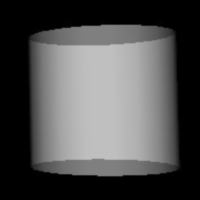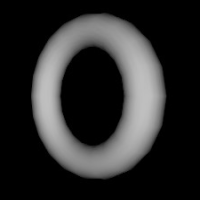Depth sorting off: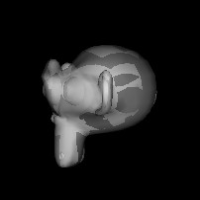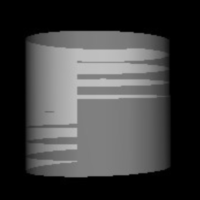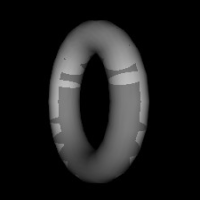The example below shows how to apply a vl::DepthSortCallback callback to an Actor in order to sort its polygons. In the example we load an object and we display it with and without polygon sorting to hightlight the effect of the vl::DepthSortCallback callback.

The DepthSortCallback class sorts the primitives of the Geometry object bound to the Actor in which the callback is installed.

This callback in order to work requires the following conditions:

• The Actor must be bound to a Geometry
• The bound Geometry must have a Geometry::vertexArray() of type ArrayFloat3
• Sorts only DrawElementsUInt/UShort/UByte objects with primitive type: PT_POINTS, PT_LINES, PT_TRIANGLES, PT_QUADS
Remarks
• The sorting is based on the position of the vertices as specified by Geometry::vertexArray() and for obvious reasons cannot take into consideration transformations made in the vertex shader or in the geometry shader.
• The sorting is performed on a per DrawCall basis. For example, if a Geometry has 2 DrawCall A and B bound to it, then the polygons, lines or points of A will alway be rendered before the ones specified by B. For this reason avoid using Geometry with more than one DrawCall whenever possible to render transparent objects, but rather rearrange each DrawCall in its own Geometry and Actor.
• vl::DepthSortCallback cannot resolve transparency ordering when two or more transparent objects intersect each other.
• You need to use vl::DepthSortCallback only when using order-dependent blending (as for the default alpha blending settings). If for example you are using additive blending you would simply set the depth mask to `false` (vl::DepthMask) and enable the depth test (vl::EN_DEPTH_TEST), but you wouldn't need to perform any sorting.

[From `App_PolyDepthSorting.cpp`]

class App_PolyDepthSorting: public BaseDemo
{
public:
App_PolyDepthSorting(const vl::String& filename): mFileName(filename) {}
void initEvent()
{
vl::Log::notify(appletInfo());
/* bind Transform */
mTransform_Left = new vl::Transform;
mTransform_Right = new vl::Transform;
/* define the Effect to be used */
/* enable depth test and lighting */
/* enable lighting and material properties */
/* enable alpha blending */
vl::ref<vl::Geometry> geom_no_sort;
vl::ref<vl::Geometry> geom_sorted;
res_db = vl::loadResource(mFileName); if ( res_db && res_db->count<vl::Geometry>() ) geom_no_sort = res_db->get<vl::Geometry>(0);
res_db = vl::loadResource(mFileName); if ( res_db && res_db->count<vl::Geometry>() ) geom_sorted = res_db->get<vl::Geometry>(0);
if (!geom_no_sort->normalArray())
geom_no_sort->computeNormals();
if (!geom_sorted->normalArray())
geom_sorted->computeNormals();
/*
if you want you can do
geom_sorted->setDisplayListEnabled(true);
or
geom_sorted->setBufferObjectEnabled(true);
but note that in this case the DepthSortCallback will schedule an update of the BufferObject or of the display list
at every frame! This will almost centainly make the use of BufferObjects or display lists useful if not harmful, performance-wise.
*/
/* add the two objects to the scene manager */
vl::ref<vl::Actor> actor_no_sort = sceneManager()->tree()->addActor( geom_no_sort.get(), effect.get(), mTransform_Left.get() );
vl::ref<vl::Actor> actor_sort = sceneManager()->tree()->addActor( geom_sorted.get(), effect.get(), mTransform_Right.get() );
/* install the vl::DepthSortCallback that will depth-sort each primitive of the Actor upon rendering */
actor_sort->actorEventCallbacks()->push_back( new vl::DepthSortCallback );
/* compute the appropriate offset to be used in updateTransforms() */
geom_no_sort->computeBounds();
geom_sorted->computeBounds();
/* positions the two objects next to one another */
updateTransforms();
/* Position the camera to nicely see the objects in the scene.
You must call this function after having positioned your objects in the scene! */
trackball()->adjustView( sceneManager(), vl::vec3(0,0,1), vl::vec3(0,1,0), 1.0f );
}
void updateTransforms()
{
vl::real degrees = vl::Time::currentTime() * 45.0f;
vl::mat4 matrix = vl::mat4::getRotation( degrees, 0,1,0 );
mTransform_Left->setLocalMatrix( vl::mat4::getTranslation(-mOffset,0,0) * matrix );
mTransform_Right->setLocalMatrix(vl::mat4::getTranslation(+mOffset,0,0) * matrix );
}